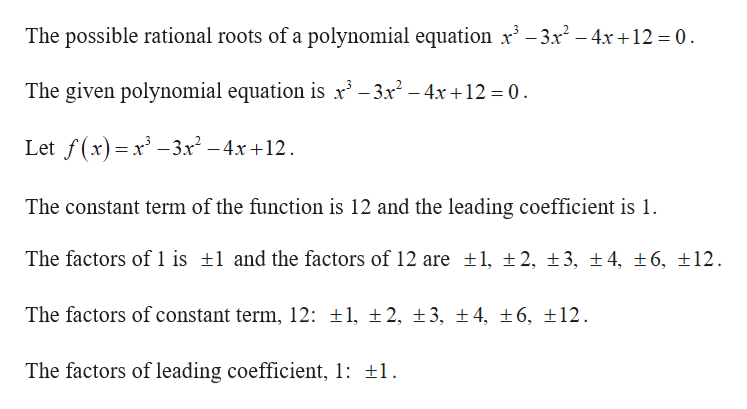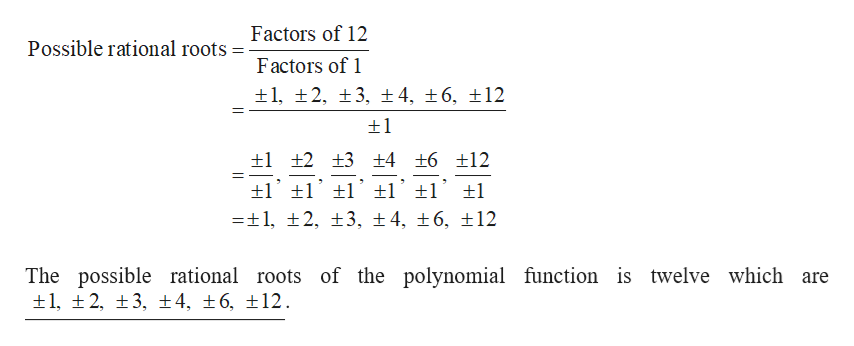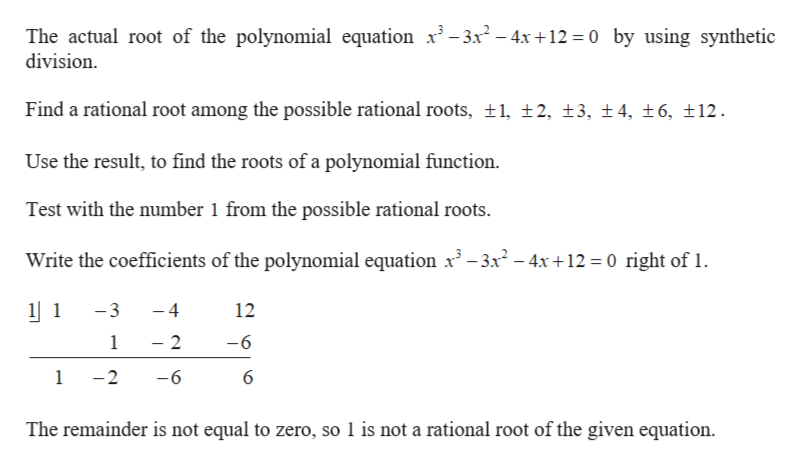Question
check_circleExpert Solution
Step 1

a. Find the possible rational roots.help_outlineImage TranscriptioncloseThe possible rational roots of a polynomial equation x3-3x2-4x+12 = 0 The given polynomial equation is x -3x2-4x +12 0 Let f(x)x-3r2-4x +12 = The constant term of the function is 12 and the leading coefficient is 1. The factors of 1 is 1 and the factors of 12 are ±1, ±2, ±3, ± 4, ±6, 12 The factors of constant term, 12: t1, 2, 3, ±4, ±6, 12 The factors of leading coefficient, 1: 1 fullscreen
Step 2

The possible rational roots are computed as follows.help_outlineImage TranscriptioncloseFactors of 12 Possible rational roots Factors of 1 1, 2, 3, ± 4, 16, 12 1 +2 +3 +4 +6 12 -1, t2, t3, ±4, 6, 12 The possible rational roots of the polynomial function is twelve which are 1, 2, 3, 4, 6, 12. fullscreen
Step 3

b. Identify the one actual r...help_outlineImage TranscriptioncloseThe actual root of the polynomial equation x3-3x- 4x+12 0 by using synthetic division Find a rational root among the possible rational roots, t1, 2, 13, t4, t 6, 12 Use the result, to find the roots of a polynomial function Test with the number 1 from the possible rational roots Write the coefficients of the polynomial equation x3 -3x2-4x +12 0 right of 1 1 -3 -4 12 1 2 -6 -6 1 -2 6 The remainder is not equal to zero, so 1 is not a rational root of the given equation fullscreen

### Want to see the full answer?

See Solution

#### Want to see this answer and more?

Solutions are written by subject experts who are available 24/7. Questions are typically answered within 1 hour*

See Solution
*Response times may vary by subject and question
Tagged in

### Algebra